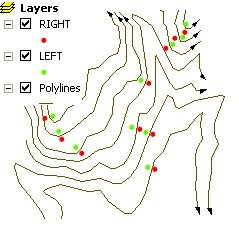## Points Along Polylines

Running programmatically

Creates points along the polylines of the input dataset.

• The points are located on user specified relative distance from the start point of the polylines.
• The user can specify an offset distance and on which side of the polylines the offset points will be created.
• If "Both" option is selected for each polyline will be created 2 points (one on the left and one on the right side), otherwise one point per polyline will be created.

### Inputs:

• A polyline feature layer
• Relative distance along polylines. A value between 0 and 1 indicating the distance from the from point as a ratio.
• 0 indicates the start point
• 0.5 indicates a point in the middle of the polyline
• 1 indicates the end of the polyline
• Side of the points - three options are available
• Both - 2 points will be created on both sides of the polylines
• Left - one point per polyline will be created and will be located on the left side of the polylines
• Right - one point per polyline will be created and will be located on the right side of the polylines
• Offset -a distance from the polyline for the points to be created. If not specified, the points will be on the polylines

### Outputs:

• New Point feature class with one or two (depending on the Side option) points per polyline
• The attributes of the original polylines are preserved
• The following fields are added to the point attribute table
• [ET_ID] - the FID of original polylines.
• [ET_Along] - the distance from the start point of the polyline to the point created.
• [ET_Offset] - the distance of the point created to the corresponding polyline.

Notes:

• The offset is measured in the units of the spatial reference of the input dataset
• The output spatial reference is the one of the input polyline dataset

### Examples:Two Point datasets created

RIGHT:

• Side = "Right"
• Distance Along = 0.5
• Offset Distance = 50 feet

LEFT:

• Side = "Left"
• Distance Along = 0.5
• Offset Distance = 50 feet

### Running Programmatically

(Go to TOP)

Parameters

Expression Explanation
Function Name PointsAlongPolylines
<input dataset> A String representing the input layer. Must be of Polyline type.
<output dataset> A String - the full name of the output layer.
<Relative Distance> A Double representing distance between the station lines.
<Side> Required. A String -This parameter defines on which side of the polyline the station lines will be created:
• "Both"
• "Left".
• "Right"
{Offset} A Double representing the Offset of the points created from the input polylines.

### Running the function

ETGWPath used in the table below is the full path to ETGWRun.exe (E.G. "C:\Program Files\ETSpatial Techniques\ETGeo Wizards\ETGWRun.exe")

Language Syntax
Python subprocess.call( [ETGWPath, "PointsAlongPolylines", "input dataset", "output dataset", "Relative Distance", "Side", "Offset From"])
.NET using ETGWRun.exe StartInfo.FileName = ETGWPath
StartInfo.Arguments = "PointsAlongPolylines" "input dataset" "output dataset" "Relative Distance" "Side" "Offset"
.NET using ETGWOutX.dll PointsAlongPolylines(input dataset, output dataset, Relative Distance, Side, Offset)
ArcPy arcpy.PointsAlongPolylines(input dataset, output dataset , "Relative Distance", "Side", "Offset")

Notes:

• <> - required parameter

• {} - optional parameter

• See examples for Python , .NET or ArcPy

• The argument separator for StartInfo.Arguments is space. If a string might contain a space, you need to double quote it.

(Go to TOP)